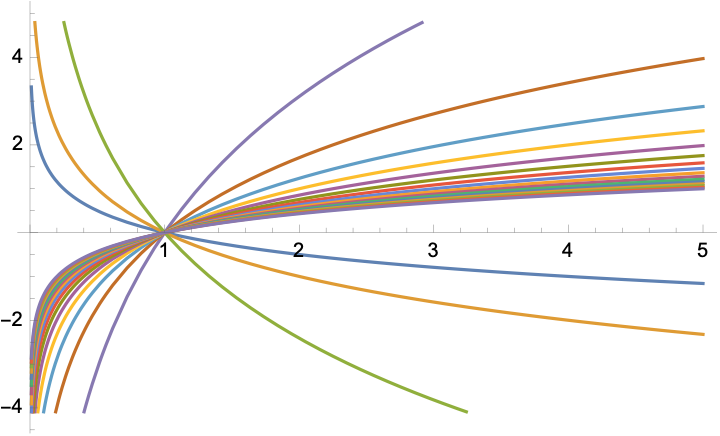# Theorems (or conjectures) for the theory of proveit.numbers.logarithms¶

In :
import proveit
# Prepare this notebook for defining the theorems of a theory:
%theorems_notebook # Keep this at the top following 'import proveit'.
from proveit import a, b, x, y
from proveit.logic import Forall, InSet, Equals, NotEquals
from proveit.numbers import (one, Add, greater, greater_eq, Less,
LessEq, Log, Real, RealPos, RealNonNeg)
from proveit.numbers.ordering import number_ordering

In :
%begin theorems

Defining theorems for theory 'proveit.numbers.logarithms'
Subsequent end-of-cell assignments will define theorems
'%end theorems' will finalize the definitions


Some other useful closure theorems should be added here. Notice the pattern of Logs, where all the Logs with positive base $a \ne 1$ go through $(1, 0)$ of course, but we have increasing functions when $a > 1$ and decreasing functions when $0 < a < 1$:In :
%end theorems

These theorems may now be imported from the theory package: proveit.numbers.logarithms